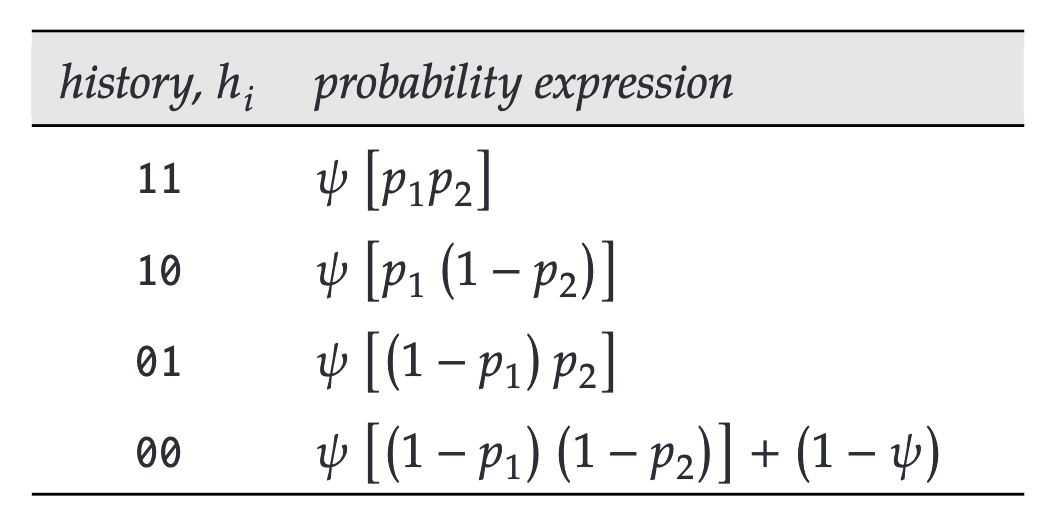Many interesting questions and applied problems in ecology and conservation require data from large spatial scales through a number of years. Often it is not possible to monitor abundance and/or vital rates across large spatial scales through time. In such cases it’s important to consider state variables other than abundance or population size that may be useful. Occupancy is one possibility that is worth considering as a useful state variable.

Occupancy is defined as the proportion of area, patches, or sample units that is occupied.

Occupancy may be the state variable of 1st choice in some cases, too, especially where there’s interest in changes in rate of occupancy through space and time.

• Studies of distribution and range, e.g., spotted owl, bull trout

• Relate patch occupancy to patch and/or landscape characteristics

When working with occupancy, it is crucial to consider the possibility that a species may be present on a site but not detected during a survey. Given this very real possibility, detection still equates to presence, but non-detection does not necessarily mean absence.

Fundamental Concept

The following quote from the introductory paragraphs of Ch. 21 of CW, which was written by Brian D. Gerber, Brittany Mosher, Daniel Martin, Larissa Bailey, and Thierry Chambert, on single-species occupancy modeling, nicely summarizes the core concepts.

When we are unable to mark animals or when the primary interest is in patterns of species occurrence or proportion of a study area that is occupied or used by a target species – occupancy models provide a flexible alternative for elucidating associations between species occurrence and the environment. Our sample unit is thus no longer an individual, but rather a ’site’, which is defined based on a study’s objective (e.g., 1km2 grid cell, wetland, habitat patch, camera-trap site, transect segment, point-count station). Occupancy models enable us to estimate the probability of occurrence of a species among sampled sites, while exploring hypotheses about factors (e.g., habitat, environmental conditions, etc.) thought to influence the species’ occurrence. The basic sampling design involves randomly selecting a set of independent sites and surveying each site multiple times (i.e., sample ‘surveys’) during a time period when the state of a site (occupied or not), does not change … . The resulting site-specific encounter histories enable us to estimate occupancy – the probability that a site is occupied– while accounting for imperfect species detection. The occupancy approach also enables us to model variation in occupancy and detection simultaneously, relative to site-specific covariates. Additional survey-specific factors can be incorporated into the detection process.

Note the following key ideas in that quote.

• evaluate hypotheses about associations between site characteristics and the probability that a species occupies a site

• learn about the detection process using multiple site visits

• estimate occupancy while accounting for imperfect detection

• model variation in occupancy and detection simultaneously

• can use site-specific characteristics
• for detection, can also use survey-specific factors

Parameters

• $$\psi$$ - occupancy probability

• $$p_i$$ - probability of detecting target species on survey $$i$$ given that the species occupies the site

• $$p^* = 1 - \displaystyle \prod_{i = 1}^{t}(1-p_{i})$$ - probability of detecting the target species at least one time (or not missing it every time) given the species occupies the site

Similar to what you did in the closed-models section of the course, you will adjust the count of sites where the species was detected ($$s_d$$) based on your ability to detect the species over $$t$$ repeated visits to $$s$$ sites: $$\hat\psi = \frac{s_d}{s \cdot \hat{p}^*}$$

Example

• $$p_i = 0.4$$
• $$t = 4$$
• $$p^* = 1 - (1-0.4)^4 = 0.8704$$
• $$s = 100$$
• $$s_d = 40$$
• $$\hat\psi = \frac{s_d}{s \cdot \hat{p}^*} = \frac{40}{100 \cdot 0.8704} = 0.46$$

Probability statements for encounter histories

For a single-state, single-species occupancy study, we’ll assume that the occupancy status of a site is closed over the course of our sampling. A site’s encounter history, which contains the outcomes for the visits to that site, are presented on a row and consists of 0’s and 1’s, where a 0 indicates that the species was not detected on a visit to a site, and 1 indicates that the species was detected.

If we know the parameters $$\psi$$ and $$p$$, we can obtain the probability of obtaining each of the possible encounter histories ($$Pr(h|\psi, p))$$. For a 2-occasion study with time-varying detection rate and a common value of occupancy for all sites, the figure below from Ch. 21 of CW presents the ($$Pr(h|\psi, p))$$.Take special note of the $$Pr(h = 00 |\psi, p))$$. Notice that there are 2 ways the history could have occurred: (1) the site was occupied but you failed to detect the species on each of your 2 visits or (2) the site could have been occupied. Because you are able to learn about $$p$$ from the sites where you detected the species on at least 1 of your visits, you can now make probabilistic statements about the occupancy status of sites with ‘00’ for their encounter history.

Likelihood example

As shown in Ch. 21 of CW, for a given model structure, you can write out the likelihood for $$\psi$$ and $$p$$ and so obtain estimates of the parameters using maximum likelihood. The example below is copied from the chapter and regards a 4-occasion study and the likelihood for a model with constant $$\psi$$ and $$p$$. By this point in the course, you should be able to work your way through the likelihood quite readily.